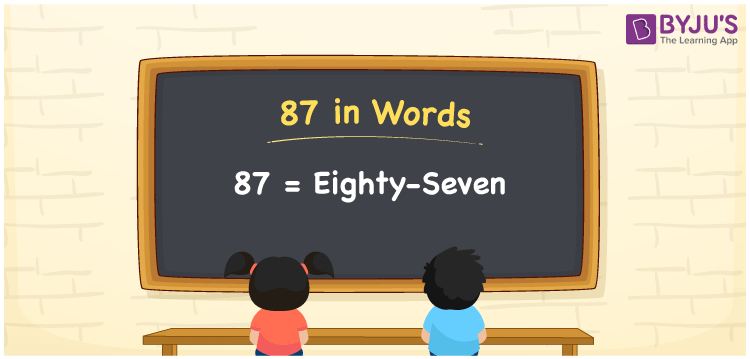# 87 in Words

87 in words is represented as Eighty-Seven. 87 is 7 more than 80. For example, Sheetal earned Rs. 87 in an hour. We can express the same sentence in words as “Sheetal earned Rupees Eighty-Seven in an hour”. We know that 87 is a cardinal number. Let us learn how to spell and write the number 87 in English in this article.

 87 in words Eighty-Seven Eighty-Seven in numerical form 87

## 87 in English Words

We know that the English alphabet is used to write numbers in words in Mathematics. Thus, 87 in words is written as Eighty-Seven.## How to Write 87 in Words?

It is vital to determine the place value of each digit of 87 while writing the number 87 in words. The place value chart for the number 87 is shown below.

 Tens Ones 8 7

Hence, the expanded form can be written as:

8 × Ten + 7 × One

= 8 x 10 + 7 x 1

= 80 + 7

= 87

= Eighty-Seven

Thus, 87 in words is written as Eighty-Seven

Interesting way of writing 87 in words

8 = Eight

7 = Seven

80 = Eighty

80 + 7 = 87

Eighty + Seven = Eighty-Seven

Hence, the word form of the number 87 is Eighty-Seven

87 is a natural number whose successor is 88 and the predecessor is 86

• 87 in words – Eighty-Seven
• Is 87 an odd number? – Yes
• Is 87 an even number? – No
• Is 87 a perfect square number? – No
• Is 87 a perfect cube number? – No
• Is 87 a prime number? – No
• Is 87 a composite number? – Yes

## Frequently Asked Questions on 87 in Words

Q1

### How to write 87 in words?

87 in words is written as Eighty-Seven.
Q2

### Simplify 70 + 17, and express in words.

Simplifying 70 + 17, we get 87. Hence, 87 in words is written as Eighty-Seven.
Q3

### 87 is a composite number. True or False.

True, 87 is a composite number.